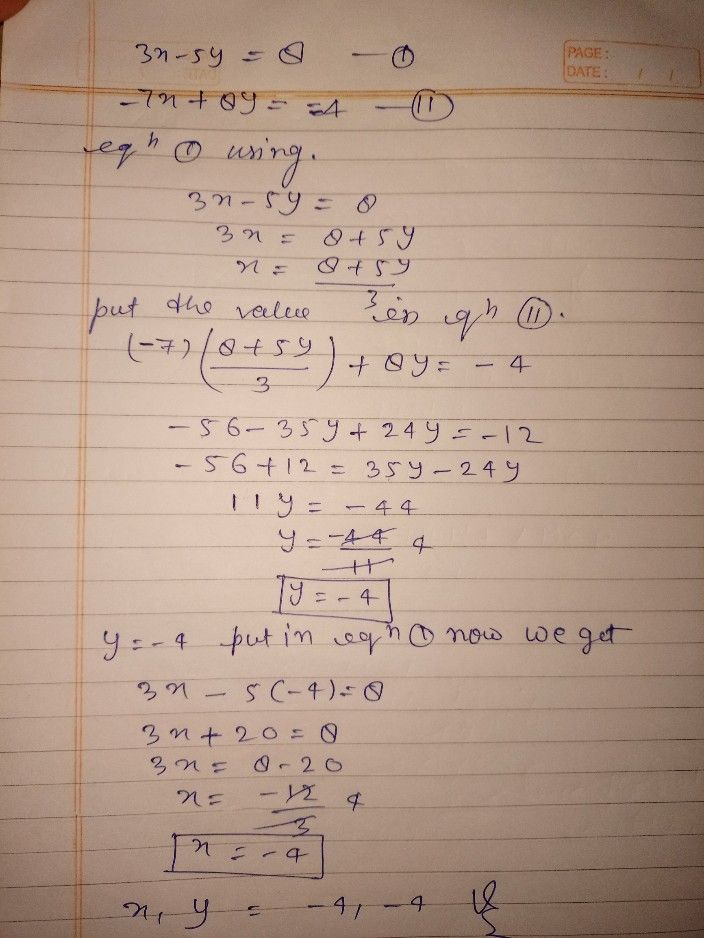Symbol
ProblemSolve the system of equations $3x-5y=8and-7x+8y=-4$ by combining the equations. $\left($ $\right)$ Submit Answer attempt i out of 2